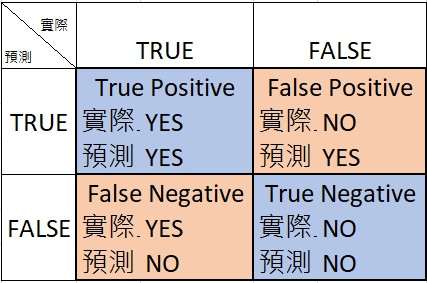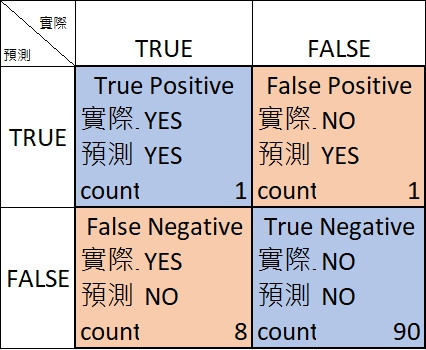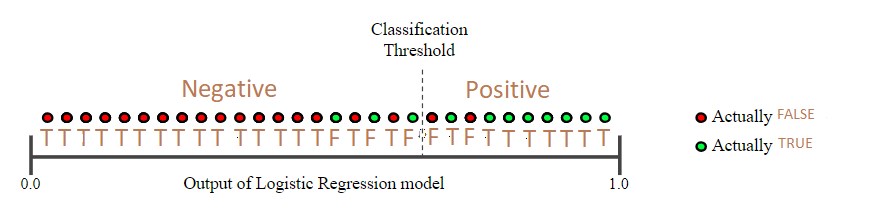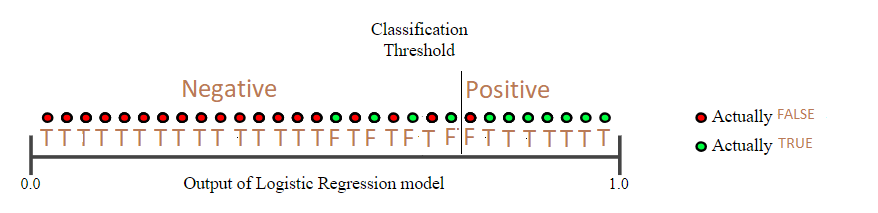2019 iT 邦幫忙鐵人賽DAY 16
0
AI & Data

[Day 16] Classification(上)

Ref.: Classification

True vs. False and Positive vs. Negative• True positive (TP): 真的有 (實際上就是有)
• False positive (FP): 假的有 (實際上就是沒有)
• False negative (FN): 假的沒有 (實際上就是有)
• True negative (TN): 真的沒有 (實際上就是沒有)

正確率Accuracy1. 它把很多實際是YES的都猜成NO ==> False positive (FN)很高
2. 或者換一個觀察，所有的examples它幾乎都猜成NO猜NO的機率高達(8+90)/100
3. 再觀察一件事：實際上是NO的比例也很高(1+90)/100=91%

Precision and Recall

Precision

What proportion of positive identifications was actually correct?

Precision = TP/(TP+FP)

Recall

What proportion of actual positives was identified correctly?

Recall = TP/(TP+FN)

Precision-Recall 拉鋸戰• Threshold右邊綠點：實際是True，猜成True ==> TP，共 8 個
• Threshold右邊紅點：實際是False，猜成True ==> FP，共 2 個
• Threshold左邊綠點：實際是True，猜成False ==> FN，共 3 個
• Threshold左邊紅點：實際是False，猜成False ==> TN，共 17 個

Precision = 8/(8+2) = 0.8
Recall = 8/(8+3) = 0.73• Threshold右邊綠點：實際是True，猜成True ==> TP，共 7 個
• Threshold右邊紅點：實際是False，猜成True ==> FP，共 1 個
• Threshold左邊綠點：實際是True，猜成False ==> FN，共 4 個
• Threshold左邊紅點：實際是False，猜成False ==> TN，共 18 個

Precision = 7/(7+1) = 0.88
Recall = 7/(7+4) = 0.64